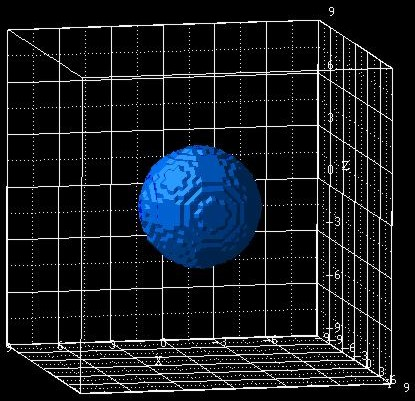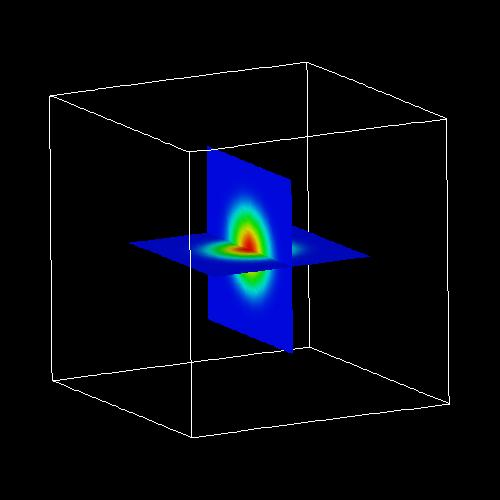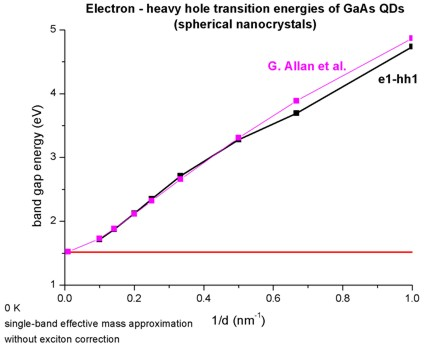nextnano.com  GUI: nextnanomat  Tool: nextnano++  Tool: nextnano³  Tool: nextnano.QCL  Download | Search | Copyright | News | Publications  * password protected nextnano³ software3D Nanocrystal

# nextnano3 - Tutorial

## 3D nanocrystals (spherical quantum dots)

Author: Stefan Birner

If you want to obtain the input files that are used within this tutorial, please contact stefan.birner@nextnano.de.
```-> 3DsphericGaAsQD_exciton_01nm.in  - ```spherical QD with a diameter of 1 nm``` -> 3DsphericGaAsQD_exciton_015nm.in - ```spherical QD with a diameter of 1.5 nm``` -> 3DsphericGaAsQD_exciton_02nm.in  - ```spherical QD with a diameter of 2 nm``` -> 3DsphericGaAsQD_exciton_03nm.in  - ```spherical QD with a diameter of 3 nm``` -> 3DsphericGaAsQD_exciton_04nm.in  - ```spherical QD with a diameter of 4 nm``` -> 3DsphericGaAsQD_exciton_05nm.in  - ```spherical QD with a diameter of 5 nm``` -> 3DsphericGaAsQD_exciton_07nm.in  - ```spherical QD with a diameter of 7 nm``` -> 3DsphericGaAsQD_exciton_10nm.in  - ```spherical QD with a diameter of 10 nm```  ```

### 3D nanocrystals (spherical quantum dots)

This tutorial aims to reproduce Figs. 1(a) and 1(b) of

Quantum confinement energies in zinc-blende III-V and group IV semiconductors
G. Allan, Y.M. Niquet, C. Delerue
Applied Physics Letters 77 (5), 639 (2000)

We consider spherical GaAs nanocrystals that are surrounded by Ga2O3 barriers.
The following figure shows a GaAs nanocrystal with a diameter of 7 nm that is surrounded by a cubic Ga2O3 matrix which has the size 18 nm x 18 nm.
The center of the spherical QD is located at (x,y,z) = (0,0,0).To keep things simple, we assume isotropic and parabolic effective masses for the electron and the heavy hole:

` conduction-band-masses = 0.067d0   0.067d0   0.067d0   ! `electron mass at Gamma conduction band```                           ...  valence-band-masses    = 0.5507d0  0.5507d0  0.5507d0  ! ```heavy hole mass```                           ...```

The heavy hole effective mass was obtained by spherically averaging over all directions:
mhh,av = 1 / ( gamma1 - 0.8 gamma2 - 1.2 gamma3 ) = 0.5507
gamma1 = 6.98
gamma2 = 2.06
gamma3 = 2.93

The following figures show an isosurface (left figure) and contour plots of 2D slices (right figure) through the ground state electron wave function (psi²) of the 7 nm spherical GaAs nanocrystal.The following figure shows the energies of the electron ground state and the heavy hole ground state in spherical GaAs nanocrystals as a function of the inverse of the nanocrystal diameter d (d = 1 nm / 1.5 nm / 3 nm / 4 nm / 5 nm / 7 nm / 10 nm).

The energies of bulk GaAs are indicated by the constant lines (Ehh = 0 eV, Ecb = 1.519 eV).This figure shows the transition energies of the electron and the heavy hole ground states in spherical GaAs nanocrystals as a function of the inverse of the nanocrystal diameter d (d = 1 nm / 1.5 nm / 3 nm / 4 nm / 5 nm / 7 nm / 10 nm).
The energy of bulk GaAs is indicated by the constant line (Egap = 1.519 eV).The transition energies can be found in this file:
`Schroedinger_1band/interband3D_qc001_vb001_sg001_cb001_sg001_deg001_dir.dat`

The following line is the relevant one where the second column shows the transition energy in units of [eV]:
```|<psi_vb001|psi_cb001_>|^2    2.123806789    0.800203758 ```
Both figures are in very good agreement with Figs. 1(a) and 1(b) of

Quantum confinement energies in zinc-blende III-V and group IV semiconductors
G. Allan, Y.M. Niquet, C. Delerue
Applied Physics Letters 77 (5), 639 (2000)

The only differences arise for very small nanocrystals having a diameter of less than 1.5 nm.

Note that the calculated values do not include the exciton correction so far.

A more realistic calculation would take into account the anisotropy of the heavy hole masses within a 6-band k.p model, or both, electron and heavy hole anisotropies within an 8-band k.p model. Finally, the exciton correction should be taken into account.

The energies for the above figures have been obtained using a 0.25 nm grid resolution which is rather coarse. The use of a finer grid is thus recommended.

### Exciton correction

The following flags are needed to include the exciton corrections:

``` \$numeric-control   ...   !---------   ! Exciton   !---------   coulomb-matrix-element                = yes  ! 'yes' or 'no'   calculate-exciton                     = yes  ! 'yes' or 'no'   exciton-iterations                    = 10   ! e.g. 5    ```(for self-consistency loop)```   exciton-residual                      = 1d-6 ! e.g. 1d-5 ```(for self-consistency loop)```   exciton-electron-state-number         = 1    ! 1 = ```electron ground state```   exciton-hole-state-number             = 1    ! 1 = ```hole ground state```   !--------------------   ! Exciton: X0   !--------------------   number-of-electron-states-for-exciton = 1    ! ```electron ground state```   number-of-hole-states-for-exciton     = 1    ! ```hole ground state

The energy of the exciton correction can be found in the file``` Schroedinger_1band/exciton_energy3D.dat ```in the column` Delta_Ex `in units of [eV].

• Please help us to improve our tutorial. Send comments to``` support [at] nextnano.com```.